# Rotations of order 2 and reflections

The remaining patterns are numbered 508, which implies that one more further question needs to the answered.

The first group are examples of patterns with containing a regular pentagon or a 10-pointed star. An example of two of these are: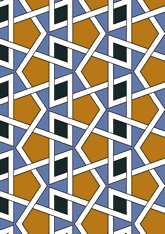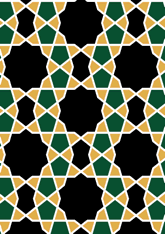The number in this group is 232, which is 45% of the total. Another question is needed - for this see page.
The second group are examples of patterns containing a regular octagon or an 8-pointed star. An example of two of these are: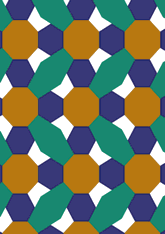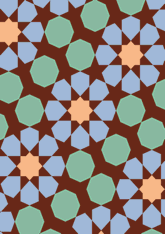The number in this group is 65, which is 12% of the total. Another question is needed - for this see page.
The third group are examples of patterns containing any regular hexagon or an 6-pointed star or a 12-pointed star. An example of two of these are: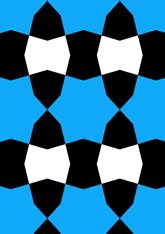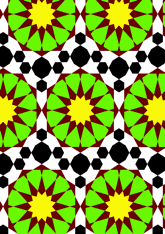The number in this group is 42, which is %42 of the total. Another question is needed - for this see page.
The last group are examples of patterns which are none of the above. An example of two of these are: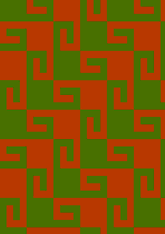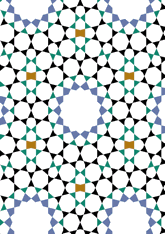The number in this group is 169, which is %169 of the total. Another question is needed - for this see page.

v53

Tiling Search was created by Brian Wichmann, and is maintained by MIT Libraries, through support from the Aga Khan Documentation Center.

Also supported by Performant Software Solutions LLC.